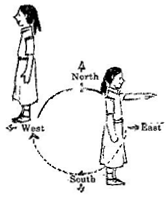### Understanding Elementary Shapes-Solutions Ex-5.2

CBSE Class –VI Mathematics
NCERT Solutions
Chapter 5 Understanding Elementary Shapes (Ex. 5.2)

Question 1. What fraction of a clockwise revolution does the hour hand of a clock turn through, when it goes from
(a) 3 to 9, (b) 4 to 7, (c) 7 to 10, (d) 12 to 9, (e) 1 to 10, (f) 6 to 3
(a) $\frac{1}{2}$ or two right angles
(b) $\frac{1}{4}$ or one right angle
(c) $\frac{1}{4}$ or one right angle
(d) $\frac{3}{4}$ or three right angles.
(e) $\frac{3}{4}$ or three right angles.
(f) $\frac{3}{4}$ or three right angles.
Question 2.Where will the hand of a clock stop if it:
(a)starts at 12 and make $\frac{1}{2}$ of a revolution, clockwise?
(b)starts at 2 and makes $\frac{1}{2}$ of a revolution, clockwise?
(c)starts at 5 and makes $\frac{1}{4}$ of a revolution, clockwise?
(d)starts at 5 and makes $\frac{3}{4}$ of a revolution, clockwise?
Answer: (a) At 6, (b) At 8, (c) At 8, (d) At 2
Question 3. Which direction will you face if you start facing:(a) East and make $\frac{1}{2}$ of a revolution clockwise?
(b) East and make 1$\frac{1}{2}$ of a revolution clockwise?
(c) West and makes $\frac{3}{4}$ of a revolution, clockwise?
(d) South and make one full revolution?
(Should we specify clockwise or anti-clockwise for this last question? Why not?)
Answer: (a) West, (b) West , (c) North, (d) South
(For answer (d), it is immaterial whether we turn clockwise or anticlockwise because one full revolution will bring us back to the original position)
Question 4. What part of a revolution have you turned through if you stand facing:
(a) East and turn clockwise to face north?
(b) South and turn clockwise to face east?
(c) West and turn clockwise to face east?
Answer: (a) $\frac{3}{4}$
(b) $\frac{3}{4}$
(c) $\frac{1}{2}$
Question 5. Find the number of right angles turned through by the hour hand of a clock when it goes from:
(a) 3 to 6, (b) 2 to 8, (c) 5 to 11, (d) 10 to 1, (e) 12 to 9, (f) 12 to 6
Answer:(a) One right angle    (b) Two right angles
(c) Two right angles      (d) One right angle
(e) Three right angles    (f) Two right angles
Question 6. How many right angles do you make if you start facing:
(a) South and turn clockwise to west?
(b) North and turn anti-clockwise to east?
(c) West and turn to west?
(d) South and turn to north?